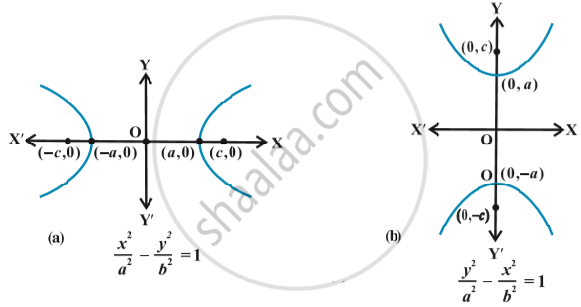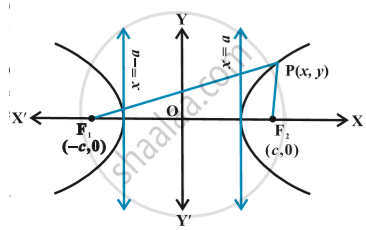# Hyperbola - Standard Equation of Hyperbola

#### notes

The equation of a hyperbola is simplest if the centre of the hyperbola is at the origin and the foci are on the x-axis or y-axis.  The two such possible orientations in following fig.Let F_1 and F_2 be the foci and O be the mid-point of the line segment
F_1F_2.  Let O be the origin and the line through O through F_2 be the positive x-axis and that through F_1 as the negative x-axis.  The line through O perpendicular to the x-axis be the y-axis.  Let the coordinates of F_1 be (– c,0) and F_2 be (c,0) in following fig.Let P(x, y) be any point on the hyperbola such that the difference of the distances from P to the farther point minus the closer point be 2a. So given,  PF_1 – PF_2 = 2a
Using the distance formula, we have
sqrt((x + c)^2+y^2) - sqrt((x-c)^2 + y^2) =2a

i.e., sqrt((x + c)^2+y^2) = 2a + sqrt((x-c)^2 + y^2)

Squaring both side, we get

(x + c)^2 + y^2 = 4a^2 + 4a  sqrt((x-c)^2 + y^2) + ((x + c)^2+y^2)

and on simplifying, we get

(cx)/a-a = sqrt((x-c)^2 + y^2)

On squaring again and further simplifying, we get

x^2/a^2 - y^2/(c^2 -a^2) = 1
i.e., x^2/a^2 -y^2/b^2=1                       (since c^2-a^2=b^2)

Hence any point on the hyperbola satisfies  x^2/a^2 -y^2/b^2=1

From the standard equations of hyperbolas, we observ that:

1. Hyperbola is symmetric with respect to both the axes, since if (x, y) is a point on the hyperbola, then (– x, y), (x, – y) and (– x, – y) are also points on the hyperbola.

2. The foci are always on the transverse axis.  It is the positive term whose denominator gives the transverse axis.

If you would like to contribute notes or other learning material, please submit them using the button below.

### Shaalaa.com

Standard Equation of Hyperbola [00:08:32]
S
0%